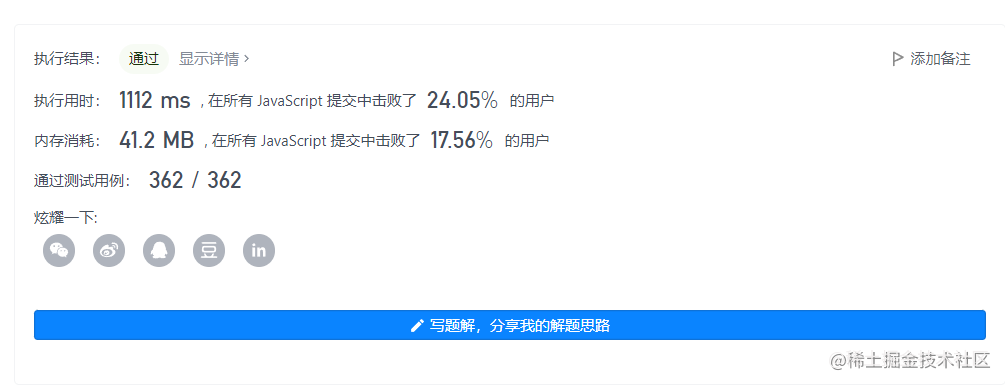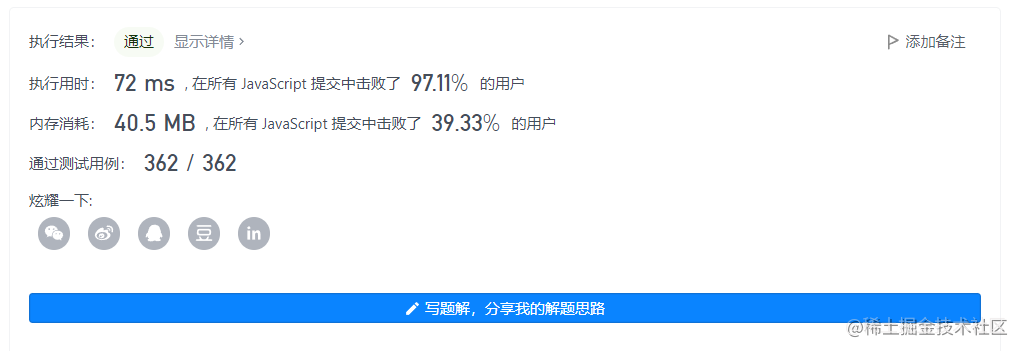2022-11-1700

1.题目
2. 解法一：使用双指针
3. 解法二：双指针优化

# 1.题目

```c```// nums 是以“引用”方式传递的。也就是说，不对实参做任何拷贝
int len = removeDuplicates(nums);

// 在函数里修改输入数组对于调用者是可见的。
// 根据你的函数返回的长度, 它会打印出数组中 该长度范围内 的所有元素。
for (int i = 0; i < len; i++) {
print(nums[i]);
}
``````

``````输入：nums = [1,1,2]

``````

``````输入：nums = [0,0,1,1,1,2,2,3,3,4]

``````

• 0 <= nums.length <= 3 * 104
• -104 <= nums[i] <= 104
• `nums` 已按升序排列

# 2. 解法一：使用双指针

```javascript```/**
* @param {number[]} nums
* @return {number}
*/
var removeDuplicates = function(nums) {
let slow = 0
let fast = 1
while(fast < nums.length) {
if(nums[slow] === nums[fast]) {
nums.splice(fast, 1)
} else {
slow = fast++
}
}
return nums.length
};
``````额，看了一下效率惨不忍睹...审题有误,看了答案后其实是不需要删除数组重复的元素的，将重复的数据往后移就好了。

# 3. 解法二：双指针优化

```javascript```/**
* @param {number[]} nums
* @return {number}
*/
/**
* @param {number[]} nums
* @return {number}
*/
var removeDuplicates = function(nums) {
let slow = 0
let fast = 1
const length = nums.length
if(length === 0) {
return 0
}
while(fast < length) {
if(nums[slow] !== nums[fast]) {
nums[++slow] = nums[fast]
}
fast++
}
return slow + 1
};
``````现在时空复杂度就好多啦~